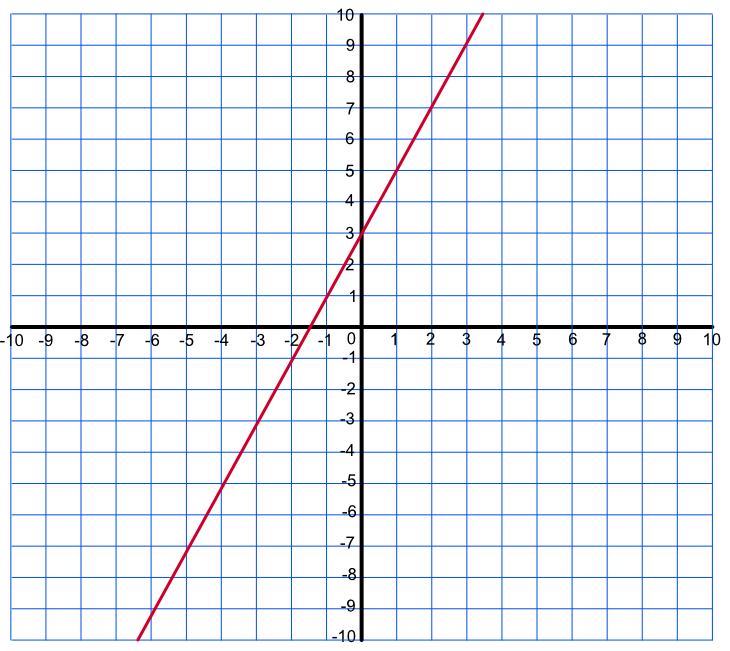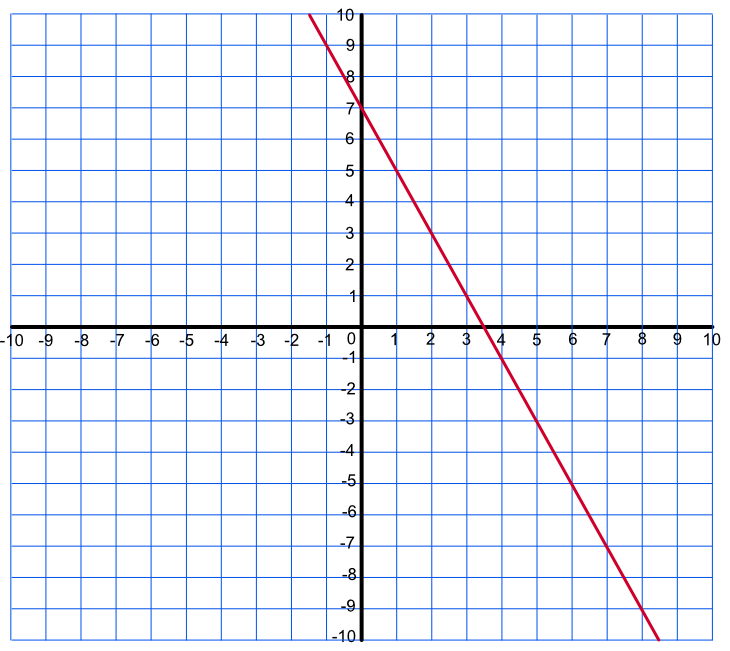Using Graphs to Solve Equations

# Using Graphs to Solve Equations

GCSE(F), GCSE(H),

Graphs can be used to estimate solutions to equations.

The solution to the equation is given where the graph crosses the x-axis. For some types of graphs, such as quadratics, there may be more than one solution if the line crosses the x-axis more than once. There may also be no solutions if the the line does not cross the x-axis at all.

When a solution is found, substitute back into the equation to check the answer.

## Examples

1. The graph shows the function 2x + 3. What is the solution to 2x + 3 = 0?Answer: x = -1.5

The line crosses the x-axis at - 1.5. Check the answer: 2(-1.5) + 3 = 0, which is true.

2. By drawing a graph, obtain the solution for -2x + 10 = 3.

Answer: x = 3.5

Rearrange the equation to -2x + 7 = 0 and plot the graph. The line crosses the x-axis at x = 3.5. Check the answer: -2(2.5) + 10 = 3, which is true.